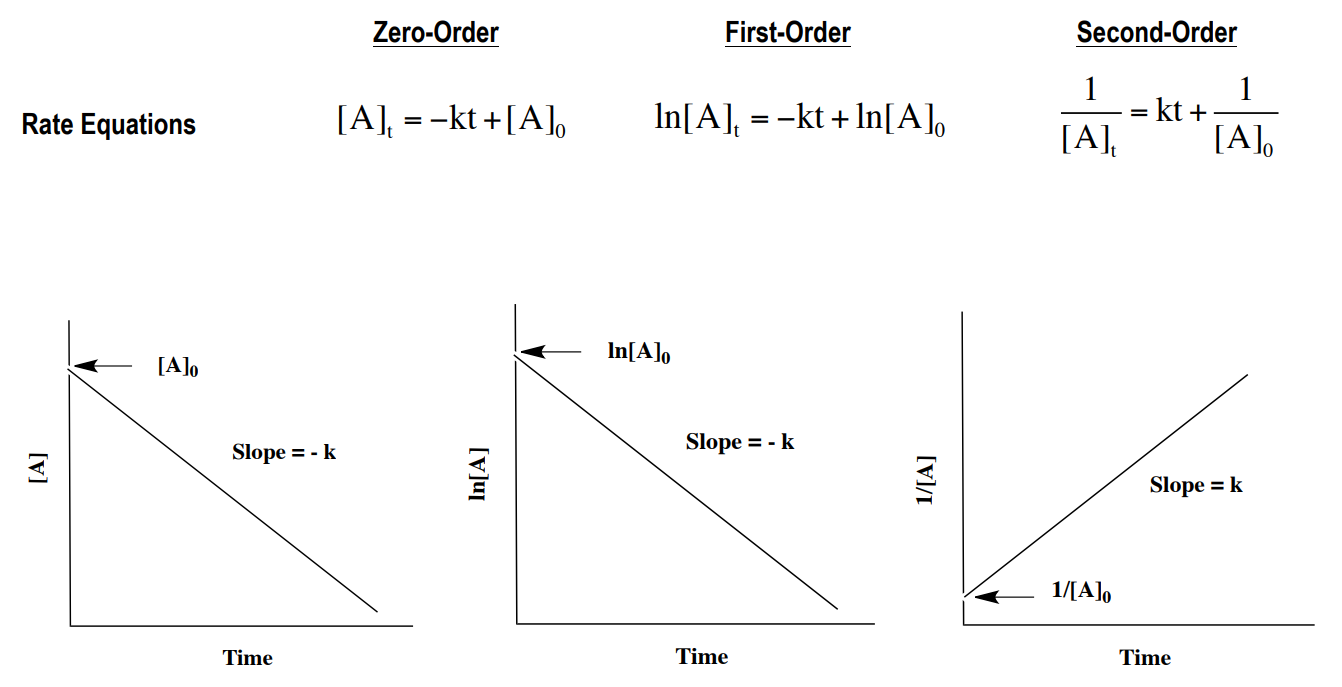# Problem: The gas-phase decomposition of NO2, NO2 (g) → 2 NO (g) + O2 (g), is studied at 383 oC, giving the following data:Time (s)[NO2] (M)0.00.1005.00.01710.00.009015.00.006220.00.0047If you used the method of initial rates to obtain the order for NO2, predict what reaction rates you would measure in the beginning of the reaction for initial concentrations of 0.200 M, 0.100 M, and 0.050 M NO2.

###### FREE Expert SolutionWe need to plot the points according to the equations above and identify which is linear.

• Since plotting on a graph can be a bit unreliable when using only pen and paper we will use the stat function of the calculator.
• We can't really see the graph but we have to calculate for R2
• An R2 that is very close to 1 (about 0.99) should mean that the plot is linear.

84% (278 ratings)###### Problem Details
The gas-phase decomposition of NO2, NO2 (g) → 2 NO (g) + O2 (g), is studied at 383 oC, giving the following data:

 Time (s) [NO2] (M) 0.0 0.100 5.0 0.017 10.0 0.0090 15.0 0.0062 20.0 0.0047

If you used the method of initial rates to obtain the order for NO2, predict what reaction rates you would measure in the beginning of the reaction for initial concentrations of 0.200 M, 0.100 M, and 0.050 M NO2.

Frequently Asked Questions

What scientific concept do you need to know in order to solve this problem?

Our tutors have indicated that to solve this problem you will need to apply the Rate Law concept. You can view video lessons to learn Rate Law. Or if you need more Rate Law practice, you can also practice Rate Law practice problems.

What professor is this problem relevant for?

Based on our data, we think this problem is relevant for Professor Lee's class at NAU.